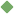factorise 1. [ verb ] (mathematics) resolve into factors, as of a polynomial Synonyms: factorize Related terms: resolve component divisor factorization To share this definition press "text" (Facebook, Twitter) or "link" (blog, mail) then paste text link Similar spelling: factor   factorize   factor_i   factoring   factorial   factor_xi   factor_ii   factor_xii   factor_vii   factor_iii   factory   factor_xiii   factor_viii   factor_x   factor_v   factor_ix   factor_iv   factor_in   factory-made   faster   factor_out